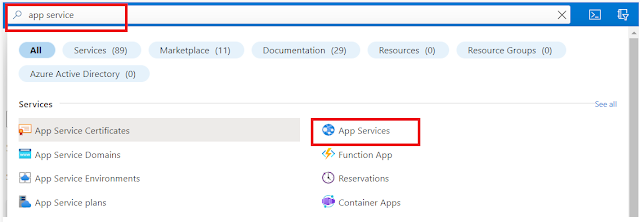### HackerRank SQL Solution - Advanced Select - Type of Triangle

• Write a query identifying the type of each record in the TRIANGLES table using its three side lengths. Output one of the following statements for each record in the table:
• Equilateral: It's a triangle with 3 sides of equal length.
• Isosceles: It's a triangle with 2 sides of equal length.
• Scalene: It's a triangle with 3 sides of differing lengths.
• Not A Triangle: The given values of A, B, and C don't form a triangle.
Input Format:
Sample Output:

``````Isosceles
Equilateral
Scalene
Not A Triangle``````
Explanation:
• Values in the tuple (20,20,23) form an Isosceles triangle, because of A = B.
• Values in the tuple (20,20,20) form an Equilateral triangle, because of A = B = C.
• Values in the tuple (20,21,22) form a Scalene triangle, because of A ≠ B ≠ C.
• Values in the tuple (13,14,30) cannot form a triangle because the combined value of sides A and B is not larger than that of side C.
Solution:

``````/* Editor - MySQL */
SELECT
CASE
WHEN A+B>C AND B+C>A AND C+A>B THEN
CASE
WHEN A=B AND B=C THEN "Equilateral"
WHEN A=B OR B=C OR A=C THEN "Isosceles"
WHEN A<>B AND B<>C AND C<>A  THEN "Scalene"
END
ELSE "Not A Triangle"
END
FROM TRIANGLES

``````
Note: The problem statement is given by hackerrank.com but the solution is generated by the Geek4Tutorial admin. We highly recommend you solve this on your own, however, you can refer to this in case of help. If there is any concern regarding this post or website, please contact us using the contact form. Thank you!